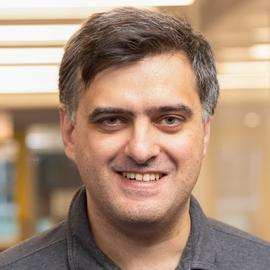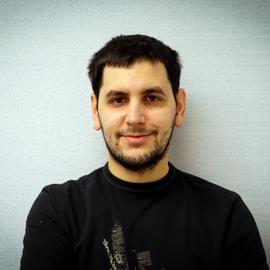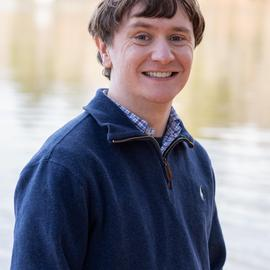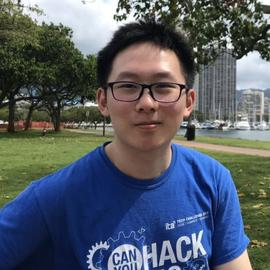The Group

ProfessorLorenzo Orecchia

Assistant ProfessorAntares Chen

University of Chicago

Local graph partitioning algorithms, applications of low-rank SDP solvers, and Statistical PhysicsErasmo Tani

University of Chicago

Vertex separators, spectral graph theory, Applications of the Burer-Monteiro framework to solving semidefinite programsKonstantinos Ameranis

University of Chicago

Algorithms, Graphs, Semidefinite Programming and optimization.Li (Alex) Wei

University of Chicago

Convex OptimizationRuimin Zhang

University of Chicago

Optimization, Learning Theory, Design and analysis of algorithms, Approximation algorithm, Online algorithmsRyan Allen Robinett

University of Chicago

Riemannian Optimization, Manifold LearningZixin Ding

University of Chicago

First order optimization algorithms interpreted under calculus of variations., Using classical mathematical or physical theory to design scalable algorithms for a broad range of optimization problems.Isabella Declue

University of Chicago

Riemannian Optimization, Manifold LearningXifan Yu

University of Chicago

Theoretical Computer Science, Design of approximation algorithms, Interplay between combinatorics, graph theory, computational complexity, and classical mathematics